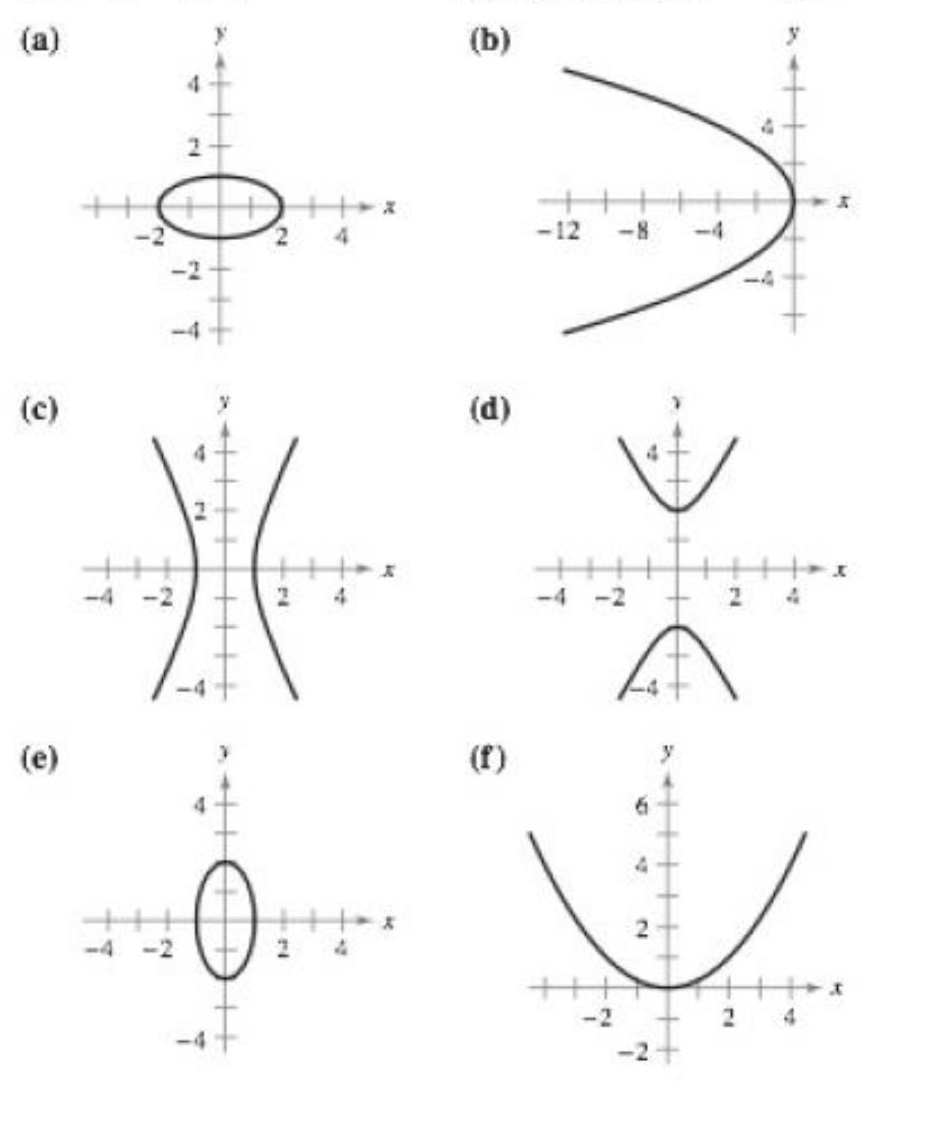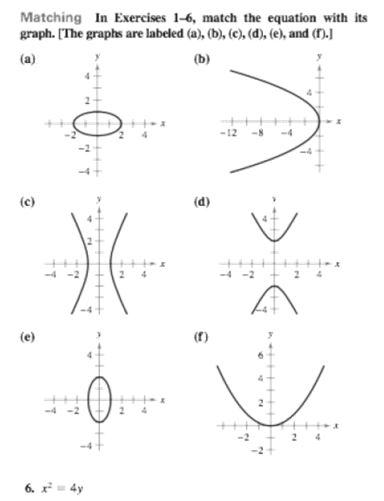Chapter 10, Problem 3RE

Chapter
Section
Textbook Problem

# Matching In Exercises 1-6, match the equation with its graph. [The graphs are labeled (a), (b), (c), (d), (e), and (f).]y 2   =   − 4 x

To determine

If the equation y2=4x matches with its graph when the options are:Explanation

Given:

The options are:

Consider the given equation y2=4x.

Here, only y has a squared term and x2 is not there. Thus, this equation represents a parabola.

In order to find whether this parabola opens horizontally or vertically, reoresent this equation in the form of standard parabola equation.

Write the equation in the standard form as:

4(x0)=(y0)2

Compare this with the standard equation of a parabola with ve

### Still sussing out bartleby?

Check out a sample textbook solution.

See a sample solution

#### The Solution to Your Study Problems

Bartleby provides explanations to thousands of textbook problems written by our experts, many with advanced degrees!

Get Started

#### In Exercises 516, evaluate the given quantity. log5125

Finite Mathematics and Applied Calculus (MindTap Course List)

#### Find f'(a). f(x)=41x

Single Variable Calculus: Early Transcendentals

#### In Problems 7-12, find the third derivative.

Mathematical Applications for the Management, Life, and Social Sciences

#### Given: m2=36mAB=4mDC Find: a)mABb)m1

Elementary Geometry for College Students